Mathematics

# Complete Guide: Abacus Level 1

974 views

 1 Introduction 2 A brief history of Abacus 3 Abacus Counting 4 Abacus Level 1 5 Summary 6 FAQs 7 External References

12 November 2020

## Introduction

Throughout history, keeping track of numbers has been problematic. Think about it, how would you keep track of numbers without pen or paper? What if you couldn’t read or write?

Without a written communication, how would you add, subtract, multiply, or divide large numbers accurately and efficiently? All of these problems are often solved with an abacus.

An abacus is a computing tool used for addition, subtraction, multiplication, and division. The abacus doesn't require a pen or paper and works for any base number system.

There are two basic sorts of the abacus, the first being a counting table, which may be a specially marked flat surface that uses small stones or beans as markers, as shown below Figure.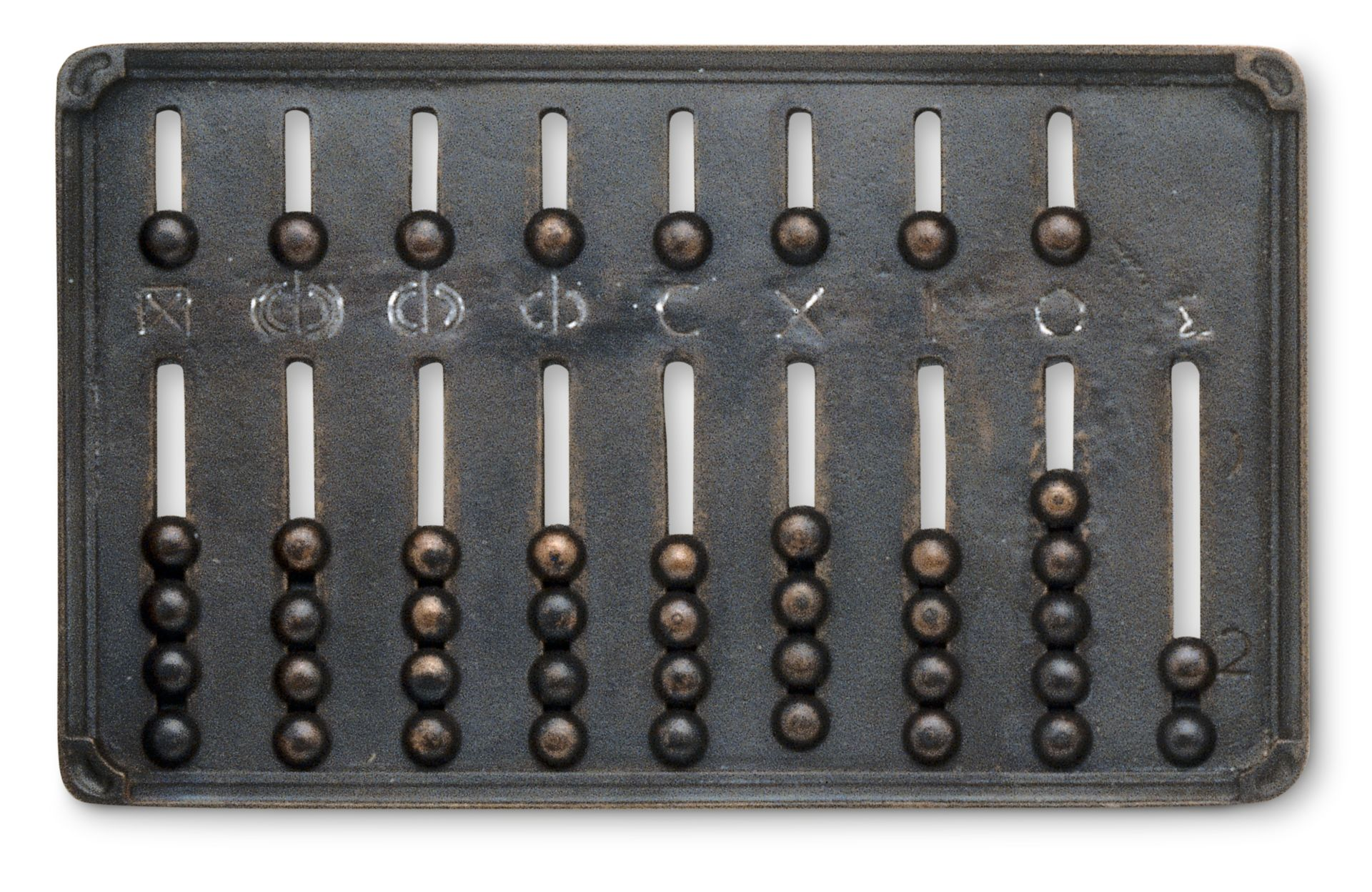The second may be a bead frame abacus, which may be a frame with beads strung on wires or rods, shown in Figure.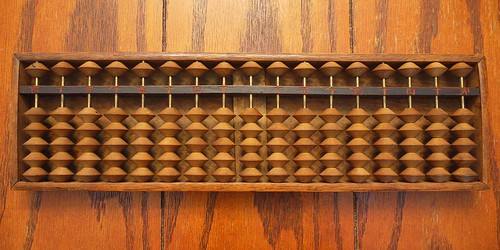The earliest documented counting boards were simple stone slabs with parallel and horizontal lines serving as place value indicators.

The normal method of calculation in Ancient Greece and Rome was done by moving counters on a smooth board or table suitably marked with lines or symbols to point out the ‘places’” (Pullan, 1968,p. 18).

The counting board’s development then stagnated for many years with the only noticeable change being the move to vertical place-values lines. The origin of the portable bead frame abacus isn't well-known.

It had been thought to have originated out necessarily for traveling merchants. Some historians credit the Chinese because the inventors of the bead frame abacus, while others believe the Romans introduced the abacus to the Chinese through trade (Moon, 1971).

From there, Russia and Japan developed their own versions of the abacus. Today the abacus lives on in rural parts of Asia and Africa and has proven to be a timeless computing tool. Its operation offers everyone the power to compute large numbers with no electrical devices.

## A brief history of Abacus

The abacus is an ancient calculator. This easy apparatus is assumed to possess originated in Babylon about 5,000 years ago. Today, the abacus remains used commonly in Japan, China, the center East, and Russia.

In China, the abacus is named a suan pan, meaning "counting tray." In Japan, it's called a soroban. Japanese school children are still taught the way to use the soroban, and competitions are held annually to seek out the foremost skillful calculators.

### Salamis Counting Board

The oldest existing counting board was discovered on the island of Salamis a few centuries ago (see Fig 3). It's been estimated that the counting board dates back to the fourth century BC (Pullan, 1968).

It’s made of white marble and measures 149 $$×$$ 75 $$×$$4.5 cm. On its top face may be a set of 11 parallel lines accompanied with Greek numerals.

Metal counters were placed on the board between the lines, and the number of counters on each line represents different values. The vertical spaces were used to indicate base units, tens, hundreds,etc., and intermediate spaces allowed the operator to mark larger values, such as fives, fifties, etc.

Tables could easily be arranged to computer addition and subtraction problems.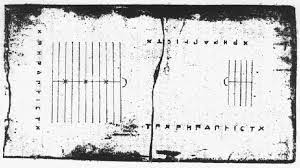To know about the detailed history of the Abacus, read Abacus: A brief history from Babylon to Japan.

## Abacus Counting

The abacus is usually constructed of varied sorts of hardwoods and comes in varying sizes. The frame of the abacus features a series of vertical rods on which a variety of wooden beads are allowed to slip freely. A horizontal beam separates the frame into two sections, referred to as the deck and therefore the third deck.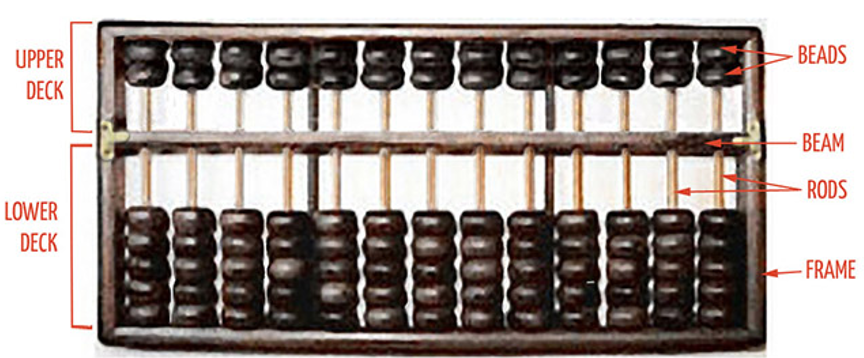Preparing The Abacus: The abacus is ready to be used ("zeroed") by placing it flat on a table and pushing all the beads on both the upper and lower decks far away from the beam by sliding the thumb along the beam.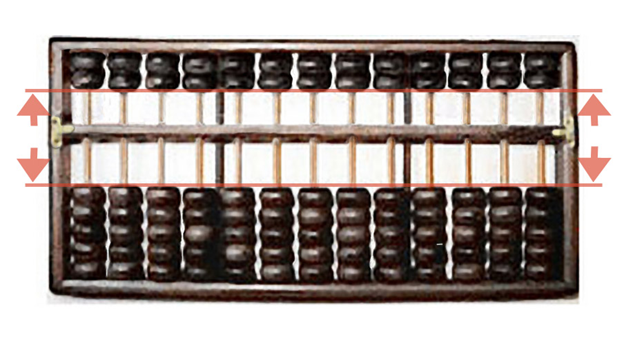Bead Values: Each bead within the deck features a value of 5; each bead within the third deck features a value of 1.

Beads are considered counted when moved towards the Beam— the piece of the abacus frame that separates the 2 decks.

After 5 beads are counted within the third deck, the results are "carried" to the deck; after both beads within the upper deck are counted, the result (10) is then carried to the left-most adjacent column.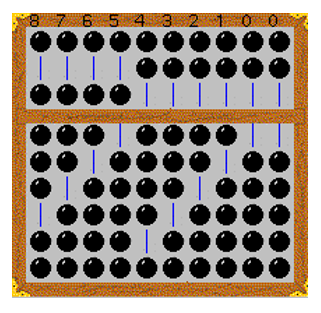The right-most column is the ones column; subsequent adjacent to the left is that the tens column; subsequent adjacent to the left is that the hundreds column, and so on.

Floating-point calculations are performed by designating an area between 2 columns because the decimal-point and every one of the rows to the proper of that space represents fractional portions while all the rows to the left represent integer digits.

The third column (from the left), representing the amount 8, is counted with 1 bead from the top-deck (value 5) and three beads from the bottom-deck (each with a worth of 1, totaling 3); the sum of the column (5+3) is 8.

Similarly, the fourth column representing the amount 7, is counted with 1 bead from the top-deck (value 5) and a couple of beads from the bottom-deck (each with a worth of 1, totaling 2); the sum of the column (5+2) is 7.

## Abacus Level 1

### How to use an Abacus for Addition?

Once you've learned the way to count Beats 1 abacus, the primary operation that we will learn additionally. There are various strategies that will be applied while learning addition.

#### The 10 Strategy

For example, if we've to feature 9+6, we'll enter 6 and 9 within the first two columns. Then moved from 6 to 9 in order that 9 becomes 10 and 6 becomes 5.

So now we will easily operate 10+5=15. Once your child masters this strategy on an abacus, you'll make them try doing it mentally.

#### The Two 5s Strategy

For example, if we've to feature 6+7, we'll enter 6 and seven within the first two wires. the 2 5s will make it 10, and that we will remain with three beads. Now performing 10+3=13 is straightforward.

This strategy works in problems where the 2 numbers being added are quite five.

Suppose we've to feature 65+89. Students will need to represent 89 on an abacus. the primary wire from the proper will have 9 and therefore the second wire will have 8. Start with the primary wire and add 5 to 9. this may end in 14. Keep the digit 4 and expire 1 to eight, thereby making it 9.

Now perform 9+6 which can end in 15. So, the result is going to be 154.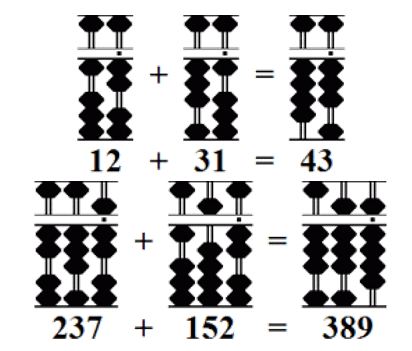### How to Use Abacus for Subtraction?

Subtraction is simply the reverse process of addition. All you would like to try to do is borrow the digits from the previous column rather than carrying them over. for instance, if you would like to subtract 867 from 932.

After entering 932 within the abacus, start subtracting column by column from the left. If you subtract 8 from 9 you'll receive 1, so you'll leave one bead within the hundreds place.

Now move to the tens place. you cannot subtract 6 from 3, so you'll need to borrow 1 within the hundreds place leaving it with 0. Now you've got to subtract 6 from 13 making it 7.

Now advance to the unit's place. Repeat the method. Because you can't subtract 7 from 2 you've got to borrow 1 from the tens place, which can convert 7 present within the tens place into 6. Now subtract 7 from 12 so you'll obtain 5.

So our final answer is going to be 932 - 867 = 65.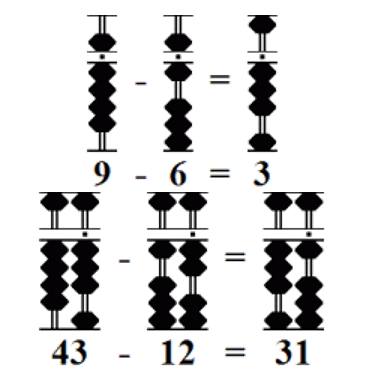### How to Use Abacus for Multiplication?

Now let's advance to the foremost important basic mathematical process which is multiplication. the way to multiply with an abacus?

To multiply small numbers, for instance, 6$$×$$4, we will ask the scholars to follow the method of addition. All they needed was to enter 6 in four different wires.

Then follow the strategy of 5, as mentioned above. So now they need to perform 5+5+5+5 = 20 and 1+1+1+1 = 4. Finally, they're going to need to add 20+4 = 24.

Well, but the above-mentioned strategy can only be utilized just in case of numbers that are small. There could also be situations wherein the scholar confronts large numbers. In those cases, we'll follow a special approach.

For example, if you're multiplying 34$$×$$12.

Step 1 - Multiply 3 with 1 then 3 with 2. Next, you'll multiply 4 with 1 then 4 with 2. Understand the pattern. This is often the part which we'll apply for all sorts of numbers.

Step 2 - Record the results of the products within the correct order. Start recording the primary product i.e. 3$$\times$$1 = 3 within the seventh wire. Next, 3$$×$$2 = 6, record it after the column during which you recorded 3 i.e. eighth wire.

Step 3- once you multiply 4$$\times$$1, add the result. Now 4+6 becomes 10.

Step 4 - Perform the last multiplication which is 4$$×$$2 = 8. Recorded within the ninth wire. So our answer is 408.

Above mentioned were a number of the foremost basic operations which may be performed using an abacus. An abacus may be a tool that will even be used for performing higher-level calculations and operations. But that is kept out of the scope of this article.

In each of the examples below beads are either added or subtracted as required.

## Summary

The abacus has played an important role in mathematics which will still be seen today. Its portability made it a well-liked tool employed by merchants and allowed it to be introduced to all parts of the planet.

Its flexibility allowed the abacus to evolve into a tool that would be used across cultures. Its mechanical operation allows addition, subtraction, multiplication, and division to be done accurately and efficiently without pencil and paper, rivaling that of a four-function calculator.

The abacus is a window into the past which allows users to process operations within the same fashion that finished thousands of years.

Moreover, the device provides students in today’s classrooms with alternatives to paper-and-pencil procedures that permit them to explore calculations during a more hands-on manner.

## Related Blogs

Cuemath, student-friendly mathematics and coding platform, conducts regular Online Live Classes for academics and skill-development, and their Mental Math App, on both iOS and Android, is a one-stop solution for kids to develop multiple skills.

Check out the fee structure for all grades and book a trial class today!

## What is an abacus?

An abacus may be a calculating instrument that uses beads that slide along a series of wires or rods set during a frame to represent the decimal places. it's the ancestor of the fashionable digital calculator. employed by merchants within the Middle Ages throughout Europe and therefore the Arabic world, it had been gradually replaced by arithmetic supported Hindu-Arabic numerals. Though rarely utilized in Europe past the 18th century, it's still utilized in the center East, China, and Japan.

## Where was Abacus first used?

The Chinese began to use counting boards, the prototype of an abacus, as early as the 4th century BC.

## What are the advantages of learning how to use an abacus?

Proficiency In Mathematics

Mathematics is taken into account to be a difficult subject for many children, though it's the foremost interesting one, if learned correctly. Learning Abacus maths results in a far better understanding of Mathematics calculations since the time when the kid is getting developed from all aspects.

Boosts Concentration And Memory

According to documented researchers, Abacus training features a positive effect on children especially when it's practiced regularly. Because the tool is visualized within the mind, it also helps in improving memory.

Enhances Logical Understanding

Over a period of time, children recall the image of an Abacus to hold out mental calculations. As he tries to reach an answer, he starts applying logic supporting the movement of the beads.

Numerical Memory

Improvement of numerical memory. Wonder how this works? An exercise to recollect two to 6 digit numbers read aloud, memorized, and orally recited would improve the numerical memory of learners. Abacus learned students were found to be much more superior within the accuracy of their memory and therefore the number of digits they were ready to hold in comparison to the non-abacus kids.

## External References

GIVE YOUR CHILD THE CUEMATH EDGE
Access Personalised Math learning through interactive worksheets, gamified concepts and grade-wise courses